# Should √2 be a Prime Number?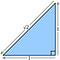From Wiki

## Does Our Prime Question Make Sense?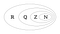From Wiki: (R) Real Numbers (left), (Q) Rational, (Z) Integer, (N) Natural numbers (right)

# √2 and the Distribution of Primes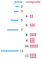From Wiki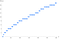Prime Counting Function From Wiki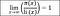Prime Number Theorem: pi(x) is the prime counting function and li(x) is the logarithmic integral function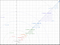Made with Geogebra: Primes are radii 2, 3, 5, and which are red, green, and blue respectively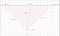Made with Geogebra: Red triangle rotated down to the x-axis

# Prime Quest Update: Where Do We Stand?

Expert Modeler | Scientist | Teacher | Engineer